Courses

# Test: Viscous Flow - 2

## 10 Questions MCQ Test Topicwise Question Bank for Mechanical Engineering | Test: Viscous Flow - 2

Description
This mock test of Test: Viscous Flow - 2 for Mechanical Engineering helps you for every Mechanical Engineering entrance exam. This contains 10 Multiple Choice Questions for Mechanical Engineering Test: Viscous Flow - 2 (mcq) to study with solutions a complete question bank. The solved questions answers in this Test: Viscous Flow - 2 quiz give you a good mix of easy questions and tough questions. Mechanical Engineering students definitely take this Test: Viscous Flow - 2 exercise for a better result in the exam. You can find other Test: Viscous Flow - 2 extra questions, long questions & short questions for Mechanical Engineering on EduRev as well by searching above.
QUESTION: 1

### At what distance r from center of a pipe of radius R the average velocity is indicated as the local velocity in laminar flow ?

Solution: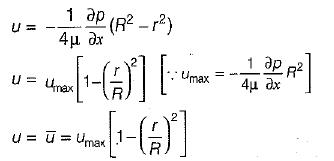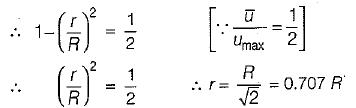QUESTION: 2

### In laminar flow through a circular pipe, the discharge varies

Solution: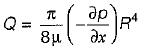So,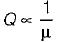Q ∝ pressure gradient and Q ∝ R4

QUESTION: 3

### For laminar flow through a long pipe, thepressure drop per unit length increases

Solution: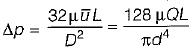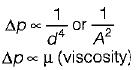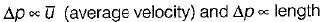QUESTION: 4

In a steady laminar flow of a given discharge of a liquid through a circular pipe of diameter d, the hydraulic gradient varies

Solution:
QUESTION: 5

The friction factor in the relation h = AfLV2/2gd for laminar flow in a circular pipe was found to be 0.01. This corresponds to a flow Reynolds number equal to

Solution:∴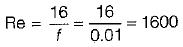QUESTION: 6

The flow profile of a fluid depends upon

Solution:

Reynold number is the factor by which type of flow is decided i.e., laminar or turbulent.

QUESTION: 7

For pipes, laminar flow occurs when reynolds number is

Solution:

For internal flow or pipe flow
(Re)Cr = 2000
i.e. Re < 2000 — Flow is laminar
Re > 2000 — Flow is turbulent

QUESTION: 8

In a two-dimensional flow of a viscous fluid, couette flow is defined for

Solution:

Coutte flow is characterized as flow of very low value of Reynolds number between two parallel

QUESTION: 9

For fully developed laminar flow through a pipe the volumetric flow is given by (symbols have the usual meaning)

Solution: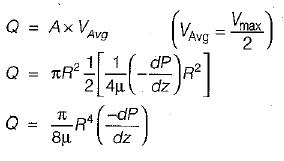QUESTION: 10

Capillary tube viscometers used for measurement of viscosity are based on

Solution: